Problem Solving Math

• Home
• Programmes
• Problem Solving Math

JEI Problem Solving Math will help students who are already advanced in
math, or who simply enjoy seeking a good challenge. The programme further
develops critical and analytical thinking skills by exposing students to
various types of complex questions. It includes math problems similar to
those found in competitions, such as America Math Competition and

Here, the focus is on reasoning, inference and problem
solving strategies, which are much-needed skills
for solving the problems.### Key Features of JEI Problem Solving Math

• 1 1

Seven major critical
thinking
domains and
two creative thinking
domains.

• 2

Develop critical thinking
and problem
solving
strategies.

• 3

Common Core
related questions.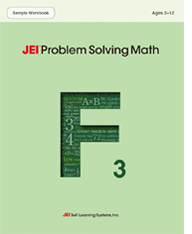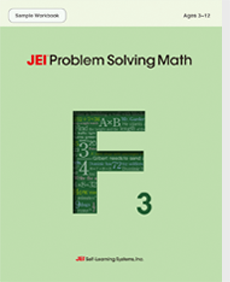View Sample

This is a sample workbook that shows various parts of the actual Problem Solving Math workbooks used at JEI.

VIEW MORELearning Objective Chart
This is a chart that shows the learning objectives for both Math and Problem Solving Math programmes.
VIEW MORE

### Problem Solving Math

Level D

• Finding the missing numbers in addition and subtraction
• Understanding number line
• Making addition and subtraction sentences
• Comparing numbers using inequality symbols
• Counting coins such as quarters, dimes, nickels, and pennies
• Composing and decomposing numbers
•••Level E

• Comparing 3-digit numbers
• Recognising unit fractions (1/2, 1/3, 1/4)
• Addition and subtraction of 2-digit and 3-digit numbers
• Inverse relationships between addition and subtraction
• Counting dollars
• Understanding symmetric figures
• Recognising polygons and their characteristics
• Telling time and reading calendars
••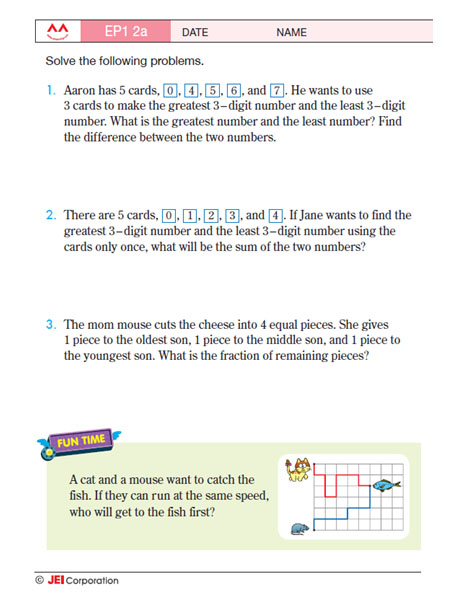•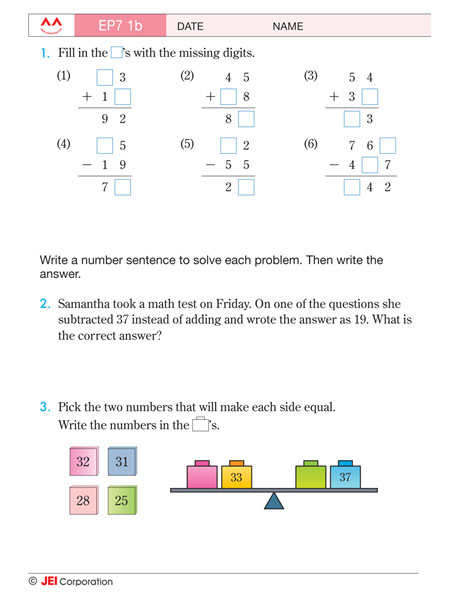Level F

• Multiplication and division of 1-digit numbers
• Making multiplication and division sentences
• Finding the amount that a fraction represents
• Addition and subtraction of 3-digit to 5-digit numbers
• Recognising rectangular prisms
••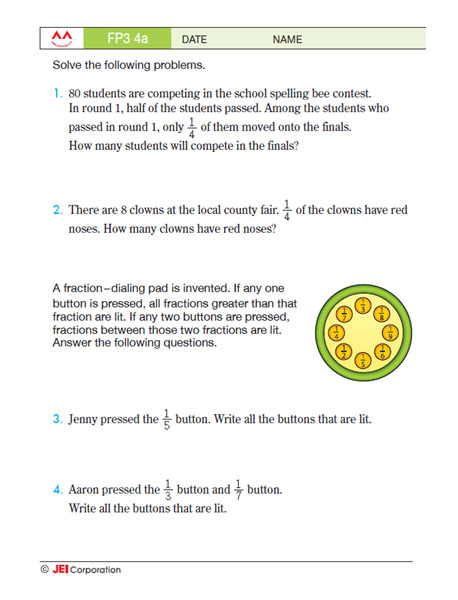•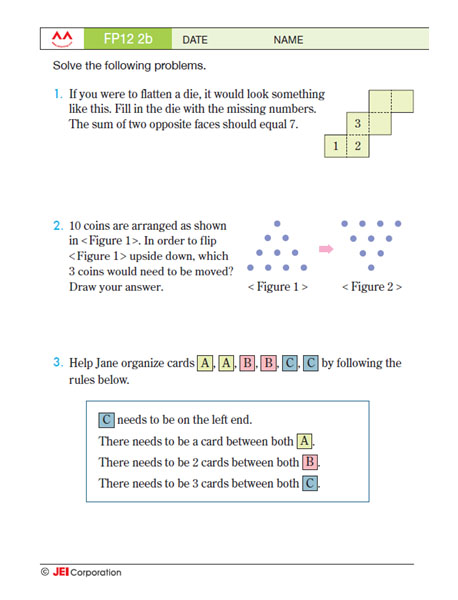Level G

• Making multiplication and division sentences
• Equivalent fractions
• Addition and subtraction of fractions and decimals
• Rate problems
• Finding perimeter of shapes
• Finding pattern of sequences and desired term from the sequences
•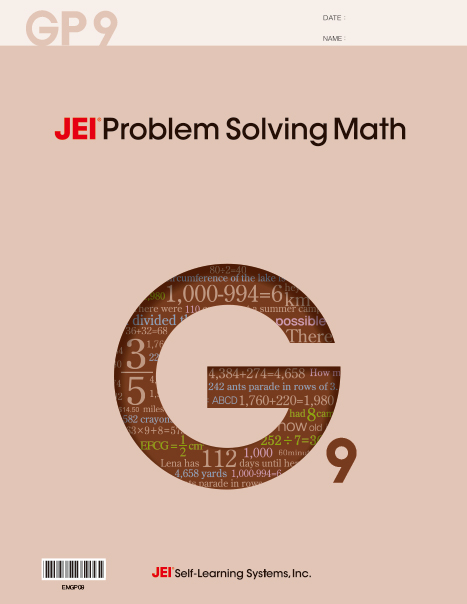••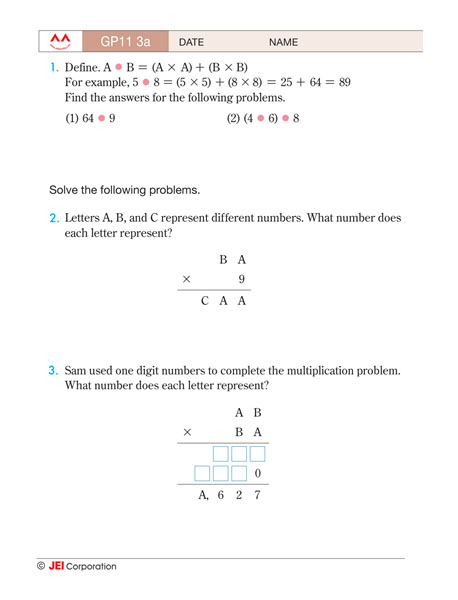Level H

• Operations and place values of numbers
• Word problems with variables
• Addition and subtraction of mixed numbers
• Word problems with fractions
• Measurement with decimals
• Calculations involving money
• Perimeter and Area of figures
• Angles formed by lines and triangles
••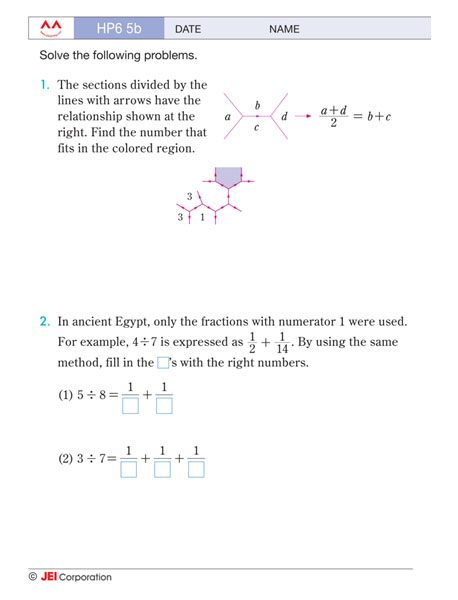•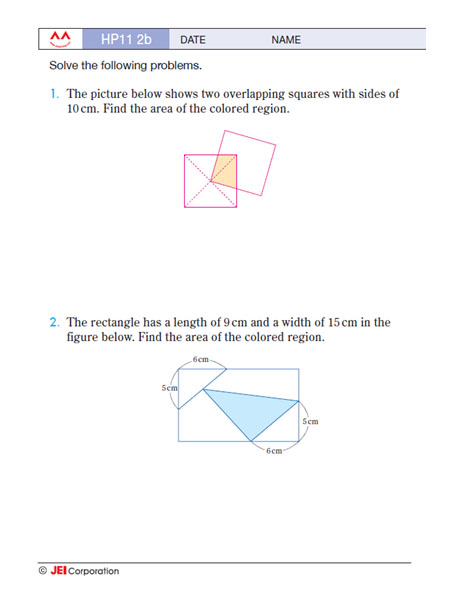Level I

• Rates and ratios
• Solving problems with percentages
• Operation of decimals
• Understanding the coordinate plane
• Evaluating expressions
• Solving equations or inequalities
• Finding surface areas and volumes
• Interpreting data using a box plot
• Finding probabilities of dependent and independent events
• Greatest common factor
• Least common multiple
•••# JEI Learning Centre International

JEI is a leader in global education and has been helping children for over 40 years in achieving academic success.

USA
JEI North American
4465 Wilshire Blvd., Suite 302,
Los Angeles, CA 90010
JEI NJ Corporate Office
440 Sylvan Ave., Suite 212,
Englewood Cliffs, NJ 07632
JEI NJ Corporate Office
440 Sylvan Ave., Suite 212,
Englewood Cliffs, NJ 07632
Hong Kong
JEI Hong Kong, LTD.
401-402, 4th Floor, Cheuk Nang Centre, No 9 Hillwood Road, Tsim Sha Tsui
India
JEI Learning Centres
8A Mohini Mohan Road Unit 1A, Siddharth Arcade Kolkata, West Bengal 700020
Australia
JEI Self-Learning Systems Pty LTD
Shop 42, Lemon Grove Shopping Centre, 427-441 Victoria Avenue, Chatswood, NSW 2067
New Zealand
JEI Self-Learning Systems Limited
Building A, 1st Floor, 20 Link Drive, Wairau Valley, Auckland 0627
Saudi Arabia
JEI Learning Centre
Mohammadyiyah Dist. Near Continetal School, 21473 Jeddah